# Magnetic Effects of Electric Current Class 10 Extra Questions with Answers Science Chapter 13

In this page, you can find CBSE Class 10 Science Chapter 13 Extra Questions and Answers Magnetic Effects of Electric Current Pdf free download, NCERT Extra Questions for Class 10 Science will make your practice complete.

## Class 10 Science Chapter 13 Extra Questions and Answers Magnetic Effects of Electric Current

Extra Questions for Class 10 Science Chapter 13 Magnetic Effects of Electric Current with Answers Solutions

### Magnetic Effects of Electric Current Extra Questions Very Short Answer Type

Question 1.
What happens if a current carrying conductor is placed in the magnetic field?
The conductor experiences a force and the direction of this force is given by Fleming’s left hand rule.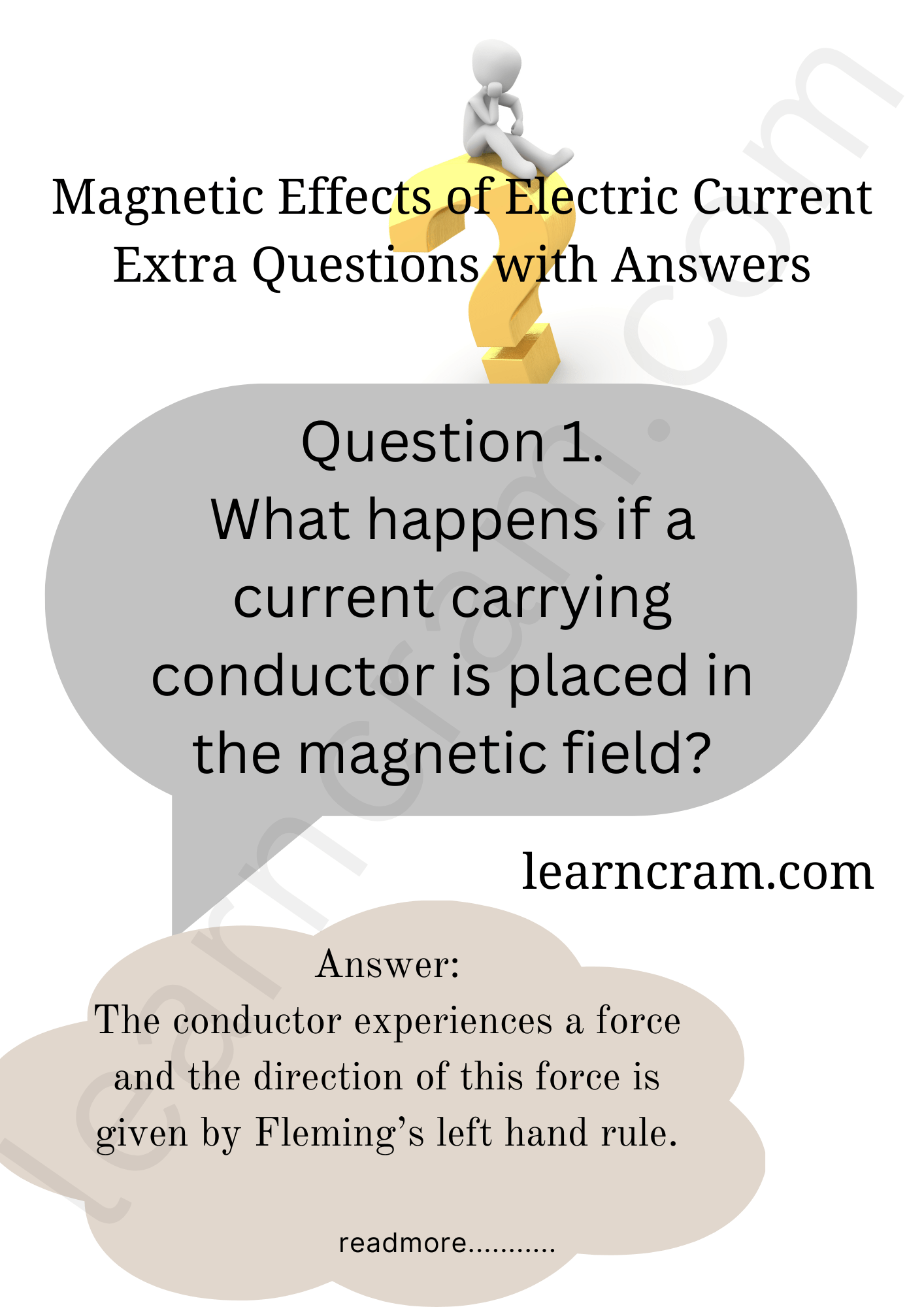Question 2.
On what effect of an electric current does an electromagnet work?
An electromagnet works on the principles of magnetic effect of current.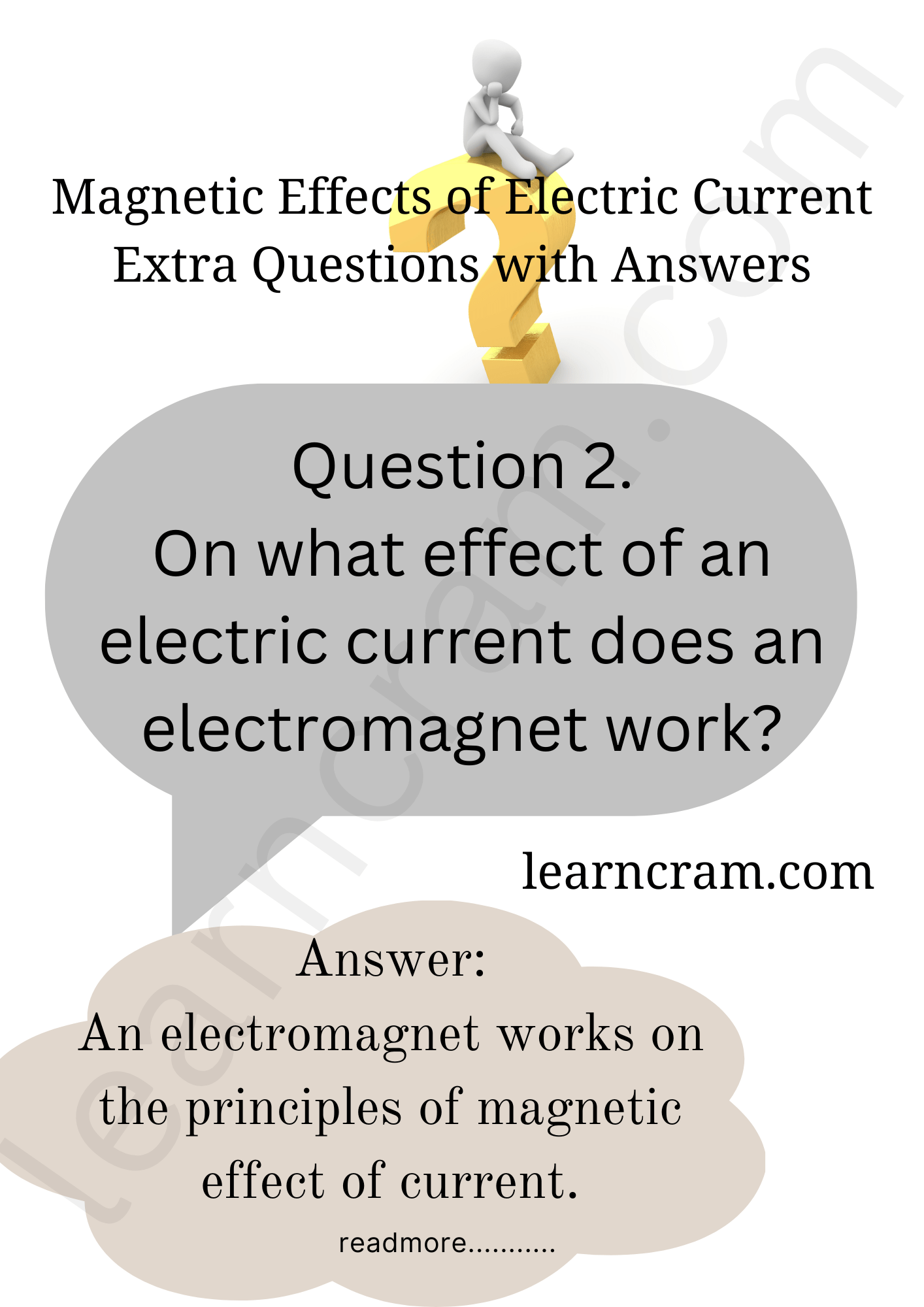Question 3.
Name the alloy which is mainly used for making permanent magnets.
The alloy ‘Alnico’ is used for making permanent magnets. It is an alloy of aluminium, nickel, cobalt and iron.

Question 4.
Why is electromagnetic induction so called?
This is due to the reason that electric current can be produced with the help of varying magnetic field without any physical contact of the source of magnetic field and the conductor.

Question 5.
Name an instrument in which the directive property of a magnet is used.
A compass needle

Question 6.
What is a solenoid?
Solenoid is a coil of many turns of wire, wrapped in the shape of a cylinder.

Question 7.
Which effect of electric current is utilised in the working of an electric fuse?
An electric fuse works on the heating effect of current.

Question 8.
What is the frequency of A.C. (alternating current) in India?
It is 50 Hz.

Question 9.
What will you do if you see a person coming in contact with a live wire?
Such a person should be provided with an insulated support like wood, plastic or rubber in order to disconnect the person from wire.

Question 10.
How can it be proved that a magnetic field exists around a current carrying metallic wire?
When a magnetic compass needle is placed close to the current carrying wire, it will get deflected.

Question 11.
How is the strength of the magnetic field at a point near a wire related to the strength of the electric current flowing in the wire?
The magnitude of magnetic field is directly proportional to the strength of the electric current flowing in the wire.

Question 12.
How is the fuse connected in an electric circuit?
Fuse is connected in series in the circuit.

Question 13.
Why is a fuse usually made of tin or tin-copper alloy?
A fuse is usually made of tin or tin-copper alloy because it has a low melting point.

Question 14.
What is the capacity of a fuse commonly used in domestic electrical fittings?
Fuses of capacity 5 ampere or 15 ampere are commonly used in domestic electrical fittings.

Question 15.
Name the device used to protect the electric circuits from overloading and short circuiting.
A fuse is used to protect circuits from overloading and short circuiting.

Question 16.
On which effect of electricity does fuse work?
Heating effect of current.

Question 17.
What kind of magnetic field is produced by a current carrying solenoid?
The magnetic field produced by a current-carrying solenoid is similar to the magnetic field produced by a bar magnet.

Question 18.
Show, with the help of a diagram, the nature of field lines of magnetic field around a current carrying straight conductor.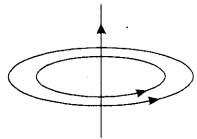Question 19.
State Faraday’s law of electromagnetic induction.
Whenever the magnetic field lines linked with a coil change due to relative motion of a magnet and the coil, an induced current is produced in the coil. The magnitude of induced current is directly proportional to the rate of change of number of magnetic field lines linked to the coil.

Question 20.
The frequency of alternating current in India is 50 Hz. What does it mean?
In India, the alternating current changes direction after every $$\frac {1}{100}$$ second, so the frequency of alternating current is 50 Hz.

Question 21.
Name the scientist who discovered the relationship between electric current and magnetic field.
Danish physicist, H.C. Oersted established the relation between electricity and magnetism.

Question 22.
What does the closeness of field lines in a magnetic field signify?
The degree of closeness of the field lines in a magnetic field indicates the strength of magnetic field in the region. The closer the field lines, the stronger is the field.

Question 23.
Name the device which converts mechanical energy into electrical energy.
Electric generator

Question 24.
Which type of generator is used at power stations?
AC generator.

Question 25.
Does the AC generator have any slip ring?
Yes, the AC generator has two slip rings.

Question 26.
What is the frequency of DC?
Zero.

### Magnetic Effects of Electric Current Extra Questions Short Answer Type

Question 1.
What is electromagnetic induction?
Whenever the magnetic flux linked with a coil changes, an induced e.m.f. (electromotive force) is produced in the coil. This phenomenon is known as electromagnetic induction and is used to generate electric current in a generator or a dynamo.

Question 2.
Write SI unit of magnetic field. Under what condition does a moving charge experience
(i) maximum force
(ii) minimum force?
The SI unit of magnetic field is tesla.
(i) Maximum force: When the velocity of charge and the magnetic field are perpendicular to each other.
(ii) Minimum force: When this charge is moving in the direction of the magnetic field.

Question 3.
What is an electromagnet?
The magnetic field produced due to current flowing in a coil or a solenoid can be used to magnetise a material like soft iron temporarily. The insulated copper wire is wrapped on a soft iron piece. When current is passed through the coil using a battery and a key the iron piece behaves like a bar magnet as long as current is being passed. Such a magnet is called an electromagnet.

Question 4.
A magnetic compass shows a deflection when placed near a current-carrying wire. How will the deflection of the compass get affected if the current in the wire is increased? Support your answer with a reason.
If the current in the wire is increased, the deflection increases. The strength of magnetic field is directly proportional to the magnitude of current passing through the straight conductor.

Question 5.
What does the divergence of magnetic field lines near the ends of a current carrying straight solenoid indicate?
The divergence, that is, the falling degree of closeness of magnetic field lines indicates the fall in strength of magnetic field near and beyond the ends of the solenoid.

Question 6.
A student performs an experiment to study the magnetic effect of current around a current carrying straight conductor with the help of a magnetic compass. He reports that
(i) the degree of deflection of the magnetic compass increases when the compass is moved away from the conductor.
(ii) the degree of deflection of the magnetic compass increases when the current through the conductor is increased.
Which of the above observation of the student appears to be wrong and why?
The first observation is wrong. Because as the distance from the conductor increases, the strength of the magnetic field will decrease. So the degree of deflection of the compass should decrease instead of increasing.

Question 7.
How does the strength of the Magnetic field at the centre of a circular coil of a wire depend on:
(b) number of turns in the coil.
(a) More the radius weakere the field.
(b) Field strength is directly proportional to the number of turns in the coil.

Question 8.
(i) How is the direction of magnetic field at a point determined?
(ii) What is the direction of magnetic field at the centre of a current-carrying circular loop?
(i) The direction of the magnetic field at a point can be found by placing a small magnetic compass at that point. The north end of the needle of a compass indicates the direction of magnetic field at a point where it is placed.
(ii) The direction of magnetic field at the centre of a current-carrying circular loop is perpendicular to the plane of the loop.

Question 9.
What is the difference between a direct current and an alternating current? How many times does AC used in India change direction in one second?
Direct current always flows in one direction but the alternating current reverses its direction periodically. Also the magnitude of current in case of DC is same throughout whereas in case of AC, it changes continuously. The frequency of AC in India is 50 Hz and in each cycle it alters direction twice. Therefore, AC changes direction 2 × 50 = 100 times in one second.

Question 10.
How can the magnitude of the induced current in a coil be increased?
The magnitude of the induced current can be increased by:

1. Increasing the number of turns in the coil.
2. Increasing the strength of the magnetic field used.
3. Increasing the rate of change of magnetic flux associated with the coil.

Question 11.
What is the role of fuse used in series with any electrical appliance? Why should a fuse with defined rating not be replaced by one with a larger rating?
Fuse is used for protecting appliances due to short-circuiting or overloading. The fuse is rated for a certain maximum current and blows off when a current more than the rated value flows through it. If a fuse is replaced by one with larger ratings, the appliances may get damaged while the protecting fuse does not burn off. This practice of using fuse of improper rating should always be avoided.

Question 12.
(i) What is the direction of magnetic field lines outside a bar-magnet?
(ii) The magnetic field lines in a given region are getting crowded. What does it indicate?
(iii) State one advantage of AC over DC.
(i) North pole to south pole.
(ii) The strength of magnetic field is higher in this region.
(iii) A.C. voltage can be stepped up and transmitted over long distances without much loss of energy.

Question 13.
What are magnetic field lines? How is the direction of a magnetic field at a point determined? Mention two important properties of magnetic field lines.
The magnetic field lines of force are the lines drawn in a magnetic field along which a hypothetical north magnetic pole would move if it is free to do so. The direction of a magnetic field at a point is in the direction of the resultant force acting on a hypothetical north pole placed at that point.

The tangent at any point on the magnetic field line gives the direction of magnetic field at that point. The direction of the magnetic field at a point can be found by placing a small magnetic compass at that point. The north end of the needle indicates the direction of the field.

Two important properties of the magnetic lines of force are:
(i) The magnetic lines of force start from north pole and terminate at south pole. Inside the magnet they travel from south pole to north pole. Thus, they are closed curves.
(ii) They do not intersect each other because at the point of intersection there will be two directions of same magnetic field which is impossible.

Question 14.
A copper coil is connected to a galvanometer. What would happen if a bar magnet is:
(i) pushed into the coil with its north pole entering first
(ii) held at rest inside the coil
(iii) pulled out again?
(i) When north pole is pushed into the coil, a momentary deflection is observed in the galvanometer. This deflection indicates that a momentary current is produced in the coil. The direction of current in the coil is anticlockwise. When seen from the end from where the magnet was pushed in.
(ii) When the magnet is held at rest, there is no deflection in the galvanometer. It indicates that no current is produced in the coil in this case.
(iii) In pulling the magnet out of the coil, a deflection in opposite direction is observed. It indicates that the current produced in the coil is in opposite direction.

Question 15.
Short circuting: Whenever live and neutral wires come in contact with each other, the incident is called short circuiting. In this case, resistance of a circuit decreases to a very small value. The decreasing of resistance increases the current. Due to this increased current, the wires get heated. This extreme heat may cause fire in the building.

Overloading: Every supply has a capacity to bear a maximum load, i.e., the power that can be supplied has a limit. Sometimes, the number of appliances which are switched on at the same time have power more than the capacity of the line. This is called overloading. In this situation, wires of the supply get heated due to extremely large current flowing through them and the circuit may catch fire. To avoid this fire, we use an electric fuse in the circuit.

Question 16.
What change in the deflection of the compass needle placed at a point near current carrying straight conductor shall be observed if the (a) current through the conductor is increased? (b) direction of current in the conductor is reversed? (c) compass is moved away from the conductor?
(a) Deflection increases.
(b) Direction of deflection is reversed.
(c) Deflection decreases.

Question 17.
Name and state the rule used for determination of direction of induced current produced in a conductor due to a changing magnetic field and give one practical application of this phenomenon in everyday life.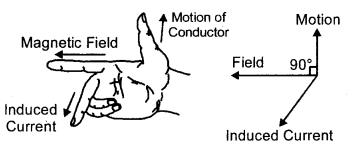Rule: Fleming’s right-hand rule.
It states that if we stretch the thumb, forefinger and middle finger of our right hand such that they are mutually perpendicular to each other. I forefinger indicates direction of magnetic field and the thumb shows the direction of motion of Induced conductor, then middle finger will show direction Current of induced current.
Application: Electrical generator.

Question 18.
A coil made of insulated copper wire is connected to a galvanometer. What will happen to the deflection of the galvanometer if this coil is moved towards a stationary bar magnet and then moved away from it? Give reason for you answer and name the phenomenon involved.
When coil is moved towards a stationary magnet, the magnetic field associated with the coil will change and so current will be induced in the coil. The causes galvanometer to show deflection in one direction. Now when coil is moved away, the magnetic field will decrease and so current induces in the opposite direction causing galvanometer to show deflection in opposite direction. The phenomenon is electromagnetic induction.

Question 19.
In what respect does the construction of an AC generator differ from that of a DC generator?
The only difference between a DC generator and an AC generator is in the way the two ends of the generator coil are connected to the outer circuit. In a DC generator, the two ends of the generator coil are connected to a split ring type commutator consisting of two half rings of copper. In an AC generator, the ends of the coil are connected to two full rings of copper called slip rings. There is no commutator in an AC generator.

### Magnetic Effects of Electric Current Extra Questions Long Answer Type

Question 1.
Briefly explain an activity to plot the magnetic field lines around a bar magnet. Sketch the field pattern for the same specifying field directions.
A region A has magnetic field lines relatively closer than another region B. Which region has stronger magnetic field. Give reason to support your answer.1. Take a drawing sheet and fix it on a smooth table with adhesive tape.
2. Place a bar magnet in the middle of the drawing sheet and draw its boundary with a sharp pencil.
3. Place a magnetic compass near one end of the magnetc (N-pole) and mark the positions of the two ends (N and S-poles) of the compass needle using a sharp pencil.
4. Shift the compass from this position and place it in such a way that S-pole of its needle is on the point you marked in previous step for N-pole.
5. Again mark the position of the other end (N-pole) of the compass needle.
6. Repeat the steps 4 and 5, till you reach the other end (S-pole) o the bar magnet.
7. Joint all the points with a sharp pencil to get a smooth curve.
8. Put the compass at some other points near the N-pole of the magnet and draw another magnetic field lines. Similarly, draw many field lines on both the sides of the bar magnet as shown in figure.
9. Observe the pattern of the magnetic field lines.

Result: Magnetic field lines can be drawn around a bar magnet using a magnetic compass. The field lines do not cross each other.
Region A has stronger magnetic field. This is due to the strength of the field is proportional to the relative closeness of field lines.

Question 2.
Draw a labelled circuit diagram of a simple electric motor and explain its working. In what way these simple electric motor are different from commercial motors?
An electric motor is a device which converts electrical energy into mechanical energy. Principle The electric motor works on the magnetic effect of current. Its principle is when a rectangular coils is placed in a magnetic field and current is passed through it, a force acts on the coil, due to which the coil rotates. When the coil rotates the shaft attached to it also rotates. The rotating shaft has mechanical energy. In this way electric energy supplied to motor is converted into mechanical energy.

Construction: An electric motor has the following parts:
1. Field Magnet: Itis a permanent U-shaped magnet NS; whose north and south poles are N and S respectively.
2. Armature: It is a rectangular coil ABCD which contains a large number of turns of insulated copper wire wound on a soft iron core. This coil is free to rotate between the pole pieces of the magnet.
3. Split Rings and Brushes: Split rings (X and Y) are simply a brass (or copper) ring which is divided into two parts. These both parts are separated from each other and are soldered to the two ends of the coil. These split rings are mounted on the shaft of the motor and can rotate with the shaft.

The split rings touch the two strips of graphite B1, B2; these strips of graphite are called the brushes. These brushes are connected to two connecting screws P and Question These brushes are fixed to the base of the motor and they keep contact with the half rings lightly. The screws are connected to terminals of battery. When current is passed by means of battery, the current enters the coil from one brush and leaves through the other.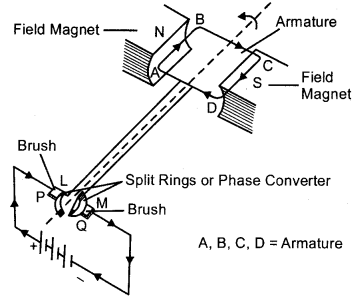Working:
Let initially coil ABCD be horizontal as shwon in Fig. When key is closed, the current begins to flowi n the coil. Initially the sides AD and BC of coil are parallel to magnetic field, so no magnetic force acts on them.

By Fleming’s left hand rule, the force on arm AB of coil is vertically downward and on side CD of coil, it is vertically upward. These two forces are equal and opposite and hence form a couple. This tends to rotate the coil in anticlockwise direction. When rotating coil becomes perpendicular to its initial position, then couple becomes zero. But due to inertia the coil Magnet continues to rotate along the same direction.

As the split rings also rotate with the coil, therefore, the split rings come in contact with other Brush brushes. [That is initially B1 had contact with X and B2 had contact with Y; now B1 has contact Split Rings or Phase Converter with has contact with X.] When this happens the direction of current in the coil is reversed.

This in turn reverses the direction of forces in AB and CD. The side of the coil will be on left hand side with a downward force on it and the side AB of coil will be on right hand side with an upward force on it. Thus a couple acts on the coil which rotates the coil in the same direction (anti-clockwise). This process is repeated again and again and the coil rotates continuously.

Due to rotation of coil, its shaft gains kinetic energy; which may be used to run electric fan, water pump, washing machine, mixer and grinder etc.

Question 3.
(i) Two circular coils P and Q are kept close to each other, of which coil P carries a current. If coil P is moved towards Q, will some current be induced in coil Q? Give reason for your answer and name the phenomenon involved.
(ii) What happens if coil P is moved away from Q?
(iii) State any two methods of inducing current in a coil.
(i) When coil P is moved towards Q, current will be induced in coil Question This is because on moving P the magnetic field associated with Q increases and so a current is induced. The phenoemenon is electromagnetic induction.
(ii) If P is moved away from Q, the field associated with Q will decrease and a current will be induced but in the opposite direction.
(iii) Current can be induced in a coil by (a) moving a magnet towards or away from the coil, (b) moving a coil towards or away from a magnet (c) rotating a coil witin a magnetic field.

Question 4.
Explain the underlying principle and working of an electric generator (or dynamo) by drawing a labelled diagram. What is the function of brushes?
Principle of electric generator. Electric generator is based on the phenomenon of electromagnetic induction i.e., a changing magnetic field in a conductor induces an electric current in the conductor. Direction of the induced current is given by Fleming’s right hand rule. In an electric generator, the magnetic field in the conductor is changed by moving the conductor in the magnetic field of the magnet.

Construction. It consists of a rotating armature ABCD containing coils of wire, pole pieces, brushes and as commutator. The two ends of the armature ABCD are connected to two metallic rings S1 and S2. The two brushes B1 and B2, which are connected to a galvanometer G, are in contact with the rings S1 and S2 respectively.
Working. in an electric generator, mechanaical work is done to turn the armature. As the coil rotates in the magnetic field, the two rings also rotate with the coil but the same brushes keep contact with the rotating rings.Fig.: Illustration of the principle of an electric generator (or Dynamo)
During the rotation of coil, its arm AB moves up and arm CD moves down cutting the magnetic lines of force, so induced current generates in the arms AB and CD in the direction A to B and C to D according to Fleming’s right hand rule. Hence, we get current flowing in the direction ABCD. As there are a number of turns in the coil, the current generated in each turn adds up to give a large current through the coil. This current will flow in the external circuit in the direction as shown in Fig.

After half a rotation, now CD starts moving up and AB moves down. As a result, the direction of induced current in the arms AB and CD changes. This gives rise to induced current in the direction DCBA and the current in the external circuit will flow in the opposite direction.

Thus, after every half a rotation, the polarity of the current in the arms AB and CD changes. Such a current which changes direction after equal intervals of time is called an alternating current. Since this electric generator producing alternating current, it is also called A.C. generator.

Function of the brushes: The carbon brushes are fixed while slip rings rotate along with the armature. These brushes are connected to the load through which the output is obtained.

### Magnetic Effects of Electric Current Extra Questions Numerical Type

Question 1.
A Coil of wine having area 0.9 m2 is placed in a uniform magnetic field 2 × 10-2 weber/m2. What will be the magnetic flux associated with the coil, if the plane of the coil is (i) along the magnetic field (ii) Perpendicular to the magnetic field?
Given: A = 0.9 m2, B = 2 × 10-2 Wb/m2
(i) θ = 90°, Ø = ?
Ø = BA cosθ = 2 × 10-2 × 0.9 × cos 90° – 0
(ii) θ = 0°, Ø = ?
\$ = BA cosθ = 2 × 10-2 × 0.9 × cos 0°
= 1.8 × 10-2 Wb

Question 2.
A Charge 3.2 × 10-19C moving with velocity 106 m/sec enters in a magnetic field of intensity 3 meber/m2 at are angle of 60°. Calculate the force acting on the charge. Write the necessary law for finding the direction of magnetic force acting on a moving charge particle in a magnetic field.
Given: q = 3.2 × 10-19C, v = 106 m/sec, B = 3 wb/m2
θ = 60°, F = ?
F = Bqv sinθ
= 3 × 3.2 × 10-19 × 106 × sin 60°
= 9.6 × 10-13 × $$\frac{\sqrt{3}}{2}$$ = 8.31 × 10-13 N.
Fleming’s left hand rule.

Question 3.
A current of 1 ampere is flowing through a conductor of length 1 m. It is placed in 2 N/A m magnetic field.
Calculate the force acting on the conductor when
(i) The conductor is perpendicular to the field
(ii) The conductor is parallel to the field
(iii) The conductor is mapping an angle of 60° with the direction of magnetic field.
= 2 × $$\frac{\sqrt{3}}{2}$$ = ∈3 = 1.732 N